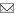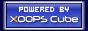Welcome Guest
www.GrammaticalPhysics.ac

Register now!
Access Detector
1 user(s) are online (1 user(s) are browsing Forum)

Members: 0
Guests: 1

more...

# What in the world is an action functional?

SourceCodeOf_HumanGenome > What in the world is an action functional? @ 2012/4/13 15:15
This problem first appeared in a message on a web forum on 2007/12/26.

This problem was proposed at JPS 2010 Spring Meeting 20pBJ-1.

This problem was discussed in my presentation material for JPS 2011 Spring Meeting 25aGC-1.

A conclusion was shown in JPS 2011 Autumn Meeting.
---
Last edited at 2012/05/01/14:51JST
SourceCodeOf_HumanGenome > To Interpret the Integrand of Feynman's Path Integral as a Solution @ 2012/4/13 15:34
Following the idea on 2012/04/11, I want to decide the potential energy functional so that the integrand of Feynman's path integral is a solution of the new grammar version of Schrödinger equation.

First I will look for a potential energy functional V such that the following functional Φ is a solution of the new grammar version of Schrödinger equation.
Φ[χ]=exp[∫dt1∫dt2 χ(t1)D(t1-t2)χ(t2)]
where D is a even function.

δΦ[χ]/δχ(t)
=[∫dt2 D(t-t2)χ(t2)+∫dt1 χ(t1)D(t1-t)]exp[∫dt1∫dt2 χ(t1)D(t1-t2)χ(t2)]
=[∫dt2 D(t-t2)χ(t2)+∫dt2 χ(t2)D(t2-t)]exp[∫dt1∫dt2 χ(t1)D(t1-t2)χ(t2)]
=2[∫dt2 D(t-t2)χ(t2)]exp[∫dt1∫dt2 χ(t1)D(t1-t2)χ(t2)] ∵D(t2-t)=D(t-t2)
=2[∫dt2 D(t-t2)χ(t2)]Φ[χ]

[δ/δχ(t)]2Φ[χ]
=2D(t-t)Φ[χ]+2[∫dt2 D(t-t2)χ(t2)][δ/δχ(t)]Φ[χ]
=2D(0)Φ[χ]+4[∫dt2 D(t-t2)χ(t2)]2Φ[χ]
=[4∫dt1∫dt2 χ(t1)D(t1-t)D(t-t2)χ(t2)+2D(0)]Φ[χ]
∵[∫dt2 D(t-t2)χ(t2)]2
=[∫dt2 D(t-t2)χ(t2)][∫dt2 D(t-t2)χ(t2)]
=∫dt1 D(t-t1)χ(t1)∫dt2 D(t-t2)χ(t2)
=∫dt1 D(t1-t)χ(t1)∫dt2 D(t-t2)χ(t2) ∵D(t-t1)=D(t1-t)
=∫dt1∫dt2 χ(t1)D(t1-t)D(t-t2)χ(t2)

∫dt [1/(2m)][(-ih/α)δ/δχ(t)]2Φ[χ]
=-[1/(2m)](h/α)2{4∫dt1∫dt2 χ(t1)[∫dt D(t1-t)D(t-t2)]χ(t2)+2∫dt D(0)}Φ[χ]
=-[1/(2m)](h/α)2{4∫dt1∫dt2 χ(t1)[∫dt D(t1-t)D(t-t2)]χ(t2)+2TD(0)}Φ[χ]
where T is the length of the circular time axis.

Φ[χ(□-ε)]=exp[∫dt1∫dt2 χ(t1-ε)D(t1-t2)χ(t2-ε)]
=exp[∫dt'1∫dt'2 χ(t'1)D((t'1+ε)-(t'2+ε))χ(t'2)] where t'1=t1-ε, t'2=t2
=exp[∫dt'1∫dt'2 χ(t'1)D(t'1-t'2)χ(t'2)]
=Φ[χ]
∴ (d/dε)Φ[χ(□-ε)]=0

To realize the equation
(ih/α)(d/dε)Φ[χ(□-ε)]|ε=0 = {∫dt [1/(2m)][(-ih/α)δ/δχ(t)]2+V[χ]}Φ[χ]=0,
the following potential energy functional V is needed.
V[χ]=[1/(2m)](h/α)2{4∫dt1∫dt2 χ(t1)[∫dt D(t1-t)D(t-t2)]χ(t2) + 2TD(0)}

D(t1-t2) → (i/h)[-(m/2)δ(2)(t1-t2)-(k/2)δ(t1-t2)]

∫dt1∫dt2 χ(t1)D(t1-t2)χ(t2)]
→ -(i/h)(m/2)∫dt1∫dt2 χ(t1(2)(t1-t2)χ(t2)-(i/h)(k/2)∫dt1∫dt2 χ(t1)δ(t1-t2)χ(t2)
=(i/h)(m/2)∫dt1∫dt2 χ(t1)[(∂/∂t1)(∂/∂t2)δ(t1-t2)]χ(t2)-(i/h)(k/2)∫dt1∫dt2 χ(t1)δ(t1-t2)χ(t2)
=(i/h)(m/2)∫dt1∫dt2 χ(1)(t1)δ(t1-t2(1)(t2)-(i/h)(k/2)∫dt1∫dt2 χ(t1)δ(t1-t2)χ(t2)
=(i/h)(m/2)∫dt χ(1)(t)χ(1)(t)-(i/h)(k/2)∫dt χ(t)χ(t)
=(i/h)∫dt{(m/2)[(d/dt)χ(t)]2-(k/2)[χ(t)]2}

Φ[χ] → exp{(i/h)∫dt[(m/2)(dχ(t)/dt)2 - (k/2)(χ(t))2]} = exp{(i/h)S[χ]}

∫dt D(t1-t)D(t-t2)
→ (i/h)2∫dt{-(m/2)δ(2)(t1-t)-(k/2)δ(t1-t)}{-(m/2)δ(2)(t-t2)-(k/2)δ(t-t2)}
=(i/h)2∫dt{(m/2)2δ(2)(t1-t)δ(2)(t-t2)+(k/2)2δ(t1-t)δ(t-t2)
+(m/2)(k/2)δ(2)(t1-t)δ(t-t2)+(k/2)(m/2)δ(t1-t)δ(2)(t-t2)}
=(i/h)2∫dt{(m/2)2δ(4)(t1-t)δ(t-t2)+(k/2)2δ(t1-t)δ(t-t2)
+(m/2)(k/2)δ(2)(t1-t)δ(t-t2)+(k/2)(m/2)δ(t1-t)δ(2)(t-t2)}
=(i/h)2{(m/2)2δ(4)(t1-t2)+(k/2)2δ(t1-t2)+(m/2)(k/2)δ(2)(t1-t2)+(k/2)(m/2)δ(2)(t1-t2)}
=(1/4)(i/h)2{m2δ(4)(t1-t2)+k2δ(t1-t2)+2mkδ(2)(t1-t2)}

V[χ]
→ [1/(2m)](h/α)2{∫dt1∫dt2 χ(t1)(i/h)2[m2δ(4)(t1-t2)+k2δ(t1-t2)
+2mkδ(2)(t1-t2)]χ(t2)+2TD(0)}
=(h/α)2(T/m)D(0)
+[1/(2m)]{-(m/α)2∫dt1∫dt2 χ(t1(4)(t1-t2)χ(t2)-(k/α)2∫dt1∫dt2 χ(t1)δ(t1-t2)χ(t2)
-2(mk/α2)∫dt1∫dt2 χ(t1(2)(t1-t2)χ(t2)}
=(h/α)2(T/m)D(0)
+[1/(2m)]{-(m/α)2∫dt[(d/dt)2χ(t)]2-(k/α)2∫dt[χ(t)]2+2(mk/α2)∫dt[(d/dt)χ(t)]2}
=(h/α)2(T/m)D(0)
-[m/(2α2)]{∫dt[(d/dt)2χ(t)]2+(k/m)2∫dt[χ(t)]2-2(k/m)∫dt[(d/dt)χ(t)]2}
=(h/α)2(T/m)D(0)
-[m/(2α2)]{∫dt[(d/dt)2χ(t)]2-2(k/m)∫dt[(d/dt)χ(t)]2+(k/m)2∫dt[χ(t)]2}
---
Last edited at 2012/05/01/15:05JST
SourceCodeOf_HumanGenome > Interaction Picture with an Action Functional as a Potential @ 2012/10/1 15:29
Reduced form of the new grammar version of Schrödinger equation tells us that an action functional is a meta potential energy.
On the other hand, as shown in 物理学正典CAN-5-1-14(in Japanese language), time developement is caused by a potential energy in the interaction picture in the old quantum mechanics.
So, I want to think meta time developement caused by an action functional.

---
Last edited at 2012/10/02/14:42JST
Site Search
Site Info
Webmasterswooder